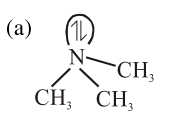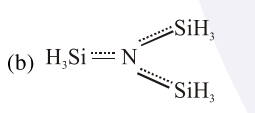# The correct statement among the following isQuestion:

The correct statement among the following is

1. $\left(\mathrm{SiH}_{3}\right)_{3} \mathrm{~N}$ is pyramidal and more basic than $\left(\mathrm{CH}_{3}\right)_{3} \mathrm{~N}$

2. $\left(\mathrm{SiH}_{3}\right)_{3} \mathrm{~N}$ is planar and more basic than $\left(\mathrm{CH}_{3}\right)_{3} \mathrm{~N}$

3. $\left(\mathrm{SiH}_{3}\right)_{3} \mathrm{~N}$ is pyramidal and less basic than $\left(\mathrm{CH}_{3}\right)_{3} \mathrm{~N}$

4. $\left(\mathrm{SiH}_{3}\right)_{3} \mathrm{~N}$ is planar and less basic than $\left(\mathrm{CH}_{3}\right)_{3} \mathrm{~N}$

Correct Option: , 4

Solution:nitrogen is $\mathrm{sp}^{3}$ hybrid and pyramidal no back-bonding i.e.more basicNitrogen $\mathrm{sp}^{2}$ hybrid and planar due to back bonding and less basic because lone pair is not available for donation.